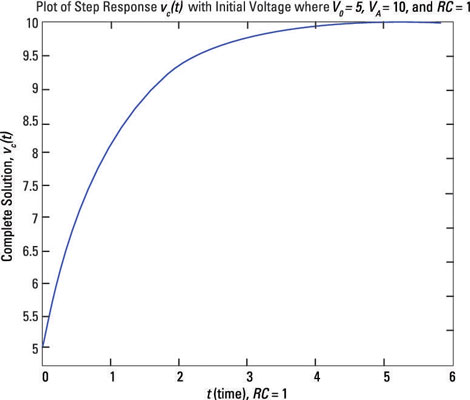##### Circuit Analysis For Dummies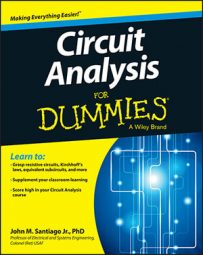After finding the zero-input response and the zero-state response of an RL parallel circuit, you can easily find the total response of the circuit. Remember that a first-order RL parallel circuit has one resistor (or network of resistors) and a single inductor.

Here is a sample RL circuit shown with zero-input response and zero-state response. The top-right diagram shows the zero-input response, which you get by setting the input to 0. The bottom-right diagram shows the zero-state response, which you get by setting the initial conditions to 0.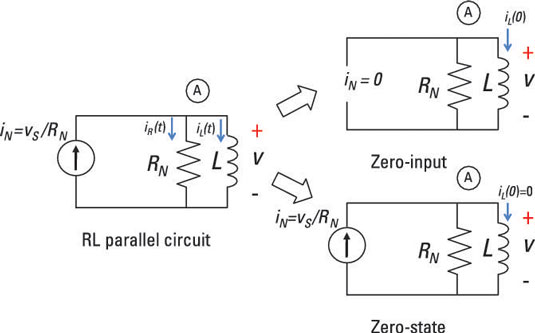The first-order differential equation you need to find the zero-input response vZI(t) for this circuit.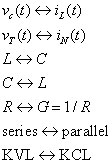After applying your math skills, you find the zero-input response of the circuit:Now to find the zero-state response, you need to study the circuit under zero initial conditions by looking at the circuit with zero inductor current at t < 0. You need to find the homogeneous and particular solutions to get the zero-state response.

After applying your math skills again, you find the zero-state response of the circuit: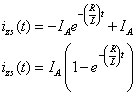To obtain the total response for the RL parallel circuit, you need to add up the two solutions, the zero-input and zero-state responses:

i(t) = iZJ(t) + iZS(t)

Substitute the zero-input and zero-state responses from the preceding sections into this equation, which gives you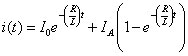Check out the total response to verify the solution i(t). When t = 0, the initial inductor current is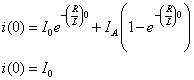This is a true statement — for sure, for sure. If you’re still not convinced, figure out when the initial condition dies out. The output should just be related to the input current or step current for this example.

After a long period of time (5 time constants), you get the following: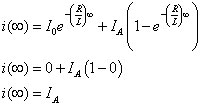The output inductor current is just the step input having a strength of IA. In other words, the inductor current reaches a value equal to the step input’s strength IA after the initial condition dies out in about 5 time constants of L/R, or 5L/R.

You see inductor currents don’t change instantaneously. With inductors, currents change gradually in going from one state to another. A parallel resistor slows things down. That’s why the time constant L/R takes into account how fast inductor currents change from one state to another.

The complete response of the inductor current follows the same shape of the capacitor voltage shown here. The shape starts at some initial current and goes to another current state after 5 time constants.